# Prime Factorization Calculator

This calculator will find all of the prime factors of a given number. Prime factorization or integer factorization of a number is the calculation of the set of prime numbers which multiply together to give the original integer. It is also known as prime decomposition.

The number 1 (one) is called a unit. It has no prime factors. When a number is a prime number (i.e., 2,3,5,7, 11), the prime factorization is just a prime number. Other numbers are called composite numbers and have a minimum of two prime factors. For repeat, a prime number is a natural number that is divisible without a remainder by itself and by a unit, not by other natural numbers.

## Math problems:

27 = 3 × 3 × 3
198 = 2 × 32 × 11
1024 = 210
19823 = 43 × 461
30258037 = 30258037

## Word problems and examples involving prime numbers

• How manyHow many different rectangles with integer page lengths have an area S = 60 cm²?
• The landThe land, which has dimensions of 220 m and 308 m, the owner wants to divide into equally large square plots with the largest possible area. How long will one side of the plot be?
• Prime factorsWrite 98 as a product of prime factors
• Reminder and quotientThere are given numbers A = 135, B = 315. Find the smallest natural number R greater than 1 so that the proportions R:A, R:B are with the remainder 1.
• Snowman 2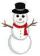On the medal, which has the shape of a circle with a diameter 18 cm is sketched snowman so that the following requirements are met: 1. snowman is composed of three circles, 2. space over snowman is the same as under it, 3. diameters of all circles express
• An exampleAn example is playfully for grade 6 from Math and I don't know how to explain it to my daughter when I don't want to use the calculator to calculate the cube root. Thus: A cuboid was made from a block of 16x18x48 mm of modeline. What will be the edge of t
• Square roomWhat is the size of the smallest square room, which can pave with tiles with dimensions 55 cm and 45 cm? How many such tiles is needed?
• Reminder and quotientThere are given the number C = 281, D = 201. Find the highest natural number S so that the C:S, D:S are with the remainder of 1,
• John SmithJohn Smith is reading a 435-page novel. If he reads the same number of pages per day and the number is a prime number greater than 10, how many pages per day will he read?
• Tiles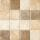It is given an area of 5m x 4m. One tile is 40 x 40 cm. How many tiles are needed in an area of 5 m x 4 m? And how many tiles need to be cut (if it not possible for the tiles to fall exactly)?
• Steps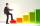Peter makes steps long 70 cm, John 45 cm long. After how many meters their footsteps meet?
• PegsFrom two sticks 240 cm and 210 cm long, it is necessary to cut the longest possible pegs for flowers so that no residues remain. How many pegs will it be?
• Oranges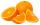Mother divided her three children's oranges in a ratio of 6:5:4. Two children gave 45 oranges. How many oranges were there?
• GroupsIn the 6th class there are 60 girls and 72 boys. We want to divide them into groups so that the number of girls and boys is the same. How many groups can you create? How many girls will be in the group?
• A ropeA rope can be cut into equal length with no rope left over. The lengths can be 15cm,18cm or 25cm. What is the shortest possible length of the rope?
• Tram stop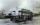At the tram stop met tram No. 4 and no. 5 at 10 AM. Tram no. 4 runs every 5 minutes, tram No. 5 at an interval of 7 minutes. How many times will meet until 12 o'clock?